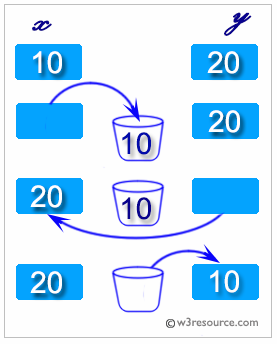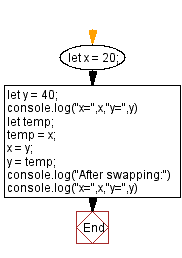# JavaScript: Swap two variables

## JavaScript Math: Exercise-55 with Solution

Write a JavaScript program to swap variables from one to another.

Swapping two variables refers to mutually exchanging the values of the variables. Generally, this is done with the data in memory.

The simplest method to swap two variables is to use a third temporary variable :
define swap(x, y)
temp := x
x := y
y := tempSample Solution:

HTML Code:

``````<!DOCTYPE html>
<html>
<meta charset="utf-8">
<title>JavaScript function to Swap two variables</title>
<body>

</body>
</html>
```
```

JavaScript Code:

``````let x = 20;
let y = 40;
console.log("x=",x,"y=",y)
let temp;
temp = x;
x = y;
y = temp;
console.log("After swapping:")
console.log("x=",x,"y=",y)
```
```

Sample Output:

```"x=" 20 "y=" 40
"After swapping:"
"x=" 40 "y=" 20
```

Flowchart:Live Demo:

See the Pen javascript-math-exercise-55 by w3resource (@w3resource) on CodePen.

Improve this sample solution and post your code through Disqus

What is the difficulty level of this exercise?

Test your Programming skills with w3resource's quiz.

﻿